# Kauffman bracket polynomial

(diff) ← Older revision | Latest revision (diff) | Newer revision → (diff)

An invariant of unoriented framed links.

It is a Laurent polynomial of one variable associated to ambient isotopy classes of unoriented framed links in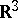(or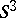), constructed by L.H. Kauffman in the summer of 1985 and denoted by. It is defined recursively as follows: For a trivial link ofcomponents, with zero framing,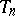, one puts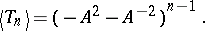For the Kauffman bracket skein triple (cf. Fig.a1) one has the Kauffman bracket skein relation: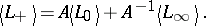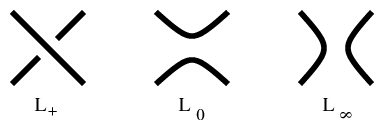Figure: k130010a

If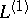is obtained fromby a positive full twist on its framing, then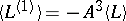. The Kauffman bracket polynomial is also considered as an invariant of regular isotopy (Reidemeister moves: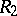,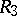, cf. Reidemeister theorem) of diagrams on the plane.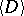is changed by the first Reidemeister move by.

The Kauffman bracket polynomial is related to a substitution of the dichromatic polynomial of signed graphs. This connection also relates the Kauffman bracket polynomial to the Potts model in statistical mechanics. State sum expansions of the dichromatic polynomial have their analogue for the Kauffman bracket polynomial. For example: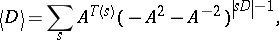where the sum is taken over all states of the link diagramand where a state codes the type of the smoothing performed at each crossing (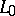or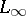type).denotes the number of smoothings of typeminus the number of smoothings of type.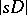denotes the number of components of the diagram after all-smoothings onare performed.

The Kauffman bracket polynomial has a straightforward generalization to the solid torus (projected onto the annulus) and to the genus-two handlebody (projected onto the disc withholes). In the first case it has values in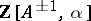and in the second case it has values in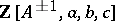, see Fig.a2.Figure: k130010b

For links in a solid torus the bracket polynomial can be used to estimate the wrapping number of the link. The wrapping conjecture says that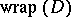for a link diagramin the annulus is equal to the-degree of[a1]. The Kauffman bracket skein module (cf. Skein module) is a generalization of the Kauffman bracket polynomial to any-dimensional manifold.

The Kauffman bracket polynomial is a variant of the Jones polynomial. If one chooses an orientationon an unoriented link diagram, then one defines an oriented link invariant, where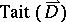is the Tait number (or writhe number) of, defined to be the sum of signs over all crossings of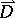. Then the Jones polynomial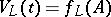for. Furthermore, the Kauffman bracket polynomial satisfies: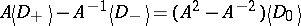and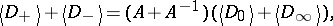and it is a specialization of both the Jones–Conway polynomial and the Kauffman polynomial.

The Kauffman bracket polynomial was essential in the proof of the Tait conjectures on alternating links. In particular, for a connected alternating diagram without a nugatory crossing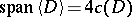, where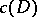is the number of crossing points of. If the diagram is prime and non-alternating, then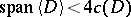.

The Kauffman bracket polynomial has several other applications, for example in the analysis of periodic links. The Reshetikhin–Turaev invariants of-manifolds can be constructed using the Kauffman bracket polynomial (via Kirby moves, cf. also Kirby calculus) [a5].

How to Cite This Entry:
Kauffman bracket polynomial. Encyclopedia of Mathematics. URL: http://encyclopediaofmath.org/index.php?title=Kauffman_bracket_polynomial&oldid=12747
This article was adapted from an original article by Jozef Przytycki (originator), which appeared in Encyclopedia of Mathematics - ISBN 1402006098. See original article